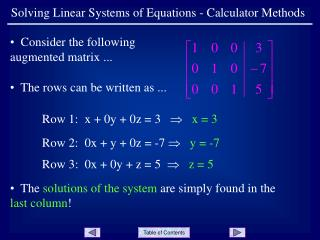# Solving Linear Systems of Equations - Calculator Methods - PowerPoint PPT PresentationDownload PresentationSolving Linear Systems of Equations - Calculator Methods

Solving Linear Systems of Equations - Calculator Methods
Download Presentation## Solving Linear Systems of Equations - Calculator Methods

- - - - - - - - - - - - - - - - - - - - - - - - - - - E N D - - - - - - - - - - - - - - - - - - - - - - - - - - -
##### Presentation Transcript

1. Solving Linear Systems of Equations - Calculator Methods • Consider the following augmented matrix ... • The rows can be written as ... Row 1: x + 0y + 0z = 3  x = 3 Row 2: 0x + y + 0z = -7  y = -7 Row 3: 0x + 0y + z = 5  z = 5 • The solutions of the system are simply found in the last column!

2. Solving Linear Systems of Equations - Calculator Methods • The augmented matrix is said to be in Reduced-Row-Echelon form, or RREF. • When solving a system of linear equations that has a unique solution, the RREF function on a calculator can be used to very quickly find the solution. Slide 2

3. Solving Linear Systems of Equations - Calculator Methods • Example 1: • Solve the system of equations • using RREF on a calculator. • Enter the augmented matrix into the calculator. See the presentation on “Matrices - Calculator Operations” is you need help with this. • Note that only columns • 2 - 4 are showing. Slide 3

4. Solving Linear Systems of Equations - Calculator Methods • Press 2nd|QUIT, then MATRIX and right arrow to MATH ... • Go down in the list to rref( which is item B, and press ENTER ... • This places rref( on the home screen. Now press MATRIX and ENTER to select matrix A ... Slide 4

5. Solving Linear Systems of Equations - Calculator Methods • Press ENTER ... • The solutions to the system are found in the last column. To show the answers as fractions, press MATH|ENTER|ENTER ... Slide 4

6. Solving Linear Systems of Equations - Calculator Methods • Another method that works nicely on the calculator to solve linear systems is in using inverse matrices. Recall that if the matrix equation ... represents a linear system, then the solution is ... Slide 4

7. Solving Linear Systems of Equations - Calculator Methods • Example 2: • Solve the system of equations • using an inverse matrix on a • calculator. • Write matrix A, the coefficients, into the calculator ... • Write matrix B, the constants, into the calculator ... Slide 4

8. Solving Linear Systems of Equations - Calculator Methods • Press 2nd Quit and then MATRIX|ENTER to place matrix A on the home screen ... • Press the x-1 key, then MATRIX|2 to place matrix B on the home screen ... • Press ENTER ... and the solutions are listed. • To write the solutions as fractions, press MATH|ENTER|ENTER ... Slide 4

9. Solving Linear Systems of Equations - Calculator Methods END OF PRESENTATION Click to rerun the slideshow.